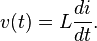# Inductor facts for kids

Kids Encyclopedia Facts

An inductor is an electrical device used in electrical circuits because of magnetic charge.

An inductor is usually made from a coil of conducting material, like copper wire, that is then wrapped around a core made from either air or a magnetic metal. If you use a more magnetic material as the core, you can get the magnetic field around the inductor to be pushed in towards the inductor, giving it better inductance. Small inductors can also be put onto integrated circuits using the same ways that are used to make transistors. Aluminum is usually used as the conducting material in this case.

## How inductors work

While a capacitor does not like changes in voltage, an inductor does not like changes in current.

In general, the relationship between the time-varying voltage v(t) across an inductor with inductance L and the time-varying current i(t) passing through it is described by the differential equation:$v(t) = L \frac{di}{dt}.$

## How inductors are used

Inductors are used often in analog circuits. Two or more inductors that have coupled magnetic flux make a transformer. Transformers are used in every power grid around the world.

Inductors are also used in electrical transmission systems, where they are used to lower the amount of voltage an electrical device gives off or lower the fault current. Because inductors are heavier than other electrical components, people have been using them in electrical equipment less often.

Inductors with an iron core are used for audio equipment, power conditioning, inverter systems, rapid transit and industrial power supplies.

## Self Inductance

We do not necessarily need two circuits in order to have inductive effects. Consider a single conducting circuit around which a current is flowing. This current generates a magnetic field which gives rise to a magnetic flux linking the circuit. We expect the flux to be directly proportional to the current, given the linear nature of the laws of magnetostatics, and the definition of magnetic flux. Thus, we can write

 (241)

where the constant of proportionality is called the self inductance of the circuit. Like mutual inductance, the self-inductance of a circuit is measured in units of henries, and is a purely geometric quantity, depending only on the shape of the circuit and number of turns in the circuit.

If the current flowing around the circuit changes by an amount in a time interval then the magnetic flux linking the circuit changes by an amount in the same time interval. According to Faraday's law, an emf

 (242)

is generated around the circuit. Since, this emf can also be written

 (243)

Thus, the emf generated around the circuit due to its own current is directly proportional to the rate at which the current changes. Lenz's law, and common sense, demand that if the current is increasing then the emf should always act to reduce the current, and vice versa. This is easily appreciated, since if the emf acted to increase the current when the current was increasing then we would clearly get an unphysical positive feedback effect in which the current continued to increase without limit. It follows, from Eq. (243), that the self-inductance of a circuit is necessarily a positive number. This is not the case for mutual inductances, which can be either positive or negative.

Consider a solenoid of length and cross-sectional area . Suppose that the solenoid has turns. When a current flows in the solenoid, a uniform axial field of magnitude

 (244)

is generated in the core of the solenoid. The field-strength outside the core is negligible. The magnetic flux linking a single turn of the solenoid is . Thus, the magnetic flux linking all turns of the solenoid is

 (245)

According to Eq. (241), the self-inductance of the solenoid is given by, which reduces to

 (246)

Note that is positive. Furthermore, is a geometric quantity depending only on the dimensions of the solenoid, and the number of turns in the solenoid.

Engineers like to reduce all pieces of electrical apparatus, no matter how complicated, to an equivalent circuit consisting of a network of just four different types of component. These four basic components are emfs, resistors, capacitors, and inductors. An inductor is simply a pure self-inductance, and is usually represented a little solenoid in circuit diagrams. In practice, inductors generally consist of short air-cored solenoids wound from enameled copper wire.

## Images for kidsInductor Facts for Kids. Kiddle Encyclopedia.